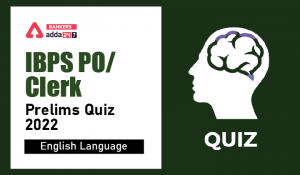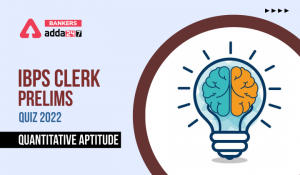Latest Banking jobs   »

# Reasoning Ability Quiz For IBPS Clerk Prelims 2022- 21st July

Directions (1-5): Read the given arrangement of numbers & symbols carefully & answer the questions based on it.

7 @ 8 5 # 4 7 1 % 2 & 8 0 1 2 > 6 7 ? 1 2 ! 5 9 3

Q1. In the given arrangement how many odd numbers are immediately followed by a symbol?
(a) One
(b) Two
(c) Three
(d) Four
(e) None of the above

Q2. In the given arrangement how many even numbers are to the left of ‘>’?
(a) Five
(b) Six
(c) Seven
(d) Eight
(e) None of the above

Q3. What will be the sum of all the numbers which are immediately preceded by a symbol?
(a) 32
(b) 33
(c) 34
(d) 35
(e) None of the above

Q4. Which of the following element is 11th to the left of 10th element from right end?
(a) 4
(b) #
(c) 5
(d) 8
(e) None of the above

Q5. If all the symbols are dropped from the above arrangement, then which of the following number will be 6th to the right of 4th from left end?
(a) 8
(b) 0
(c) 1
(d) 2
(e) None of the above

Directions (6-10): The following questions are based on the five three-digit numbers given below.

713 486 635 562 875

Q6. If the second and third digits of each number are added then the resulting sum of which number is divisible by 3?
(a) 486
(b) 713
(c) 562
(d) 875
(e) 635

Q7. If in each number second and third digits are interchanged then how many odd numbers will be formed?
(a) 2
(b) 3
(c) 5
(d) 1
(e) 4

Q8. If in each number all digits are arranged in ascending order from left to right, then which of the following will be the highest?
(a) 486
(b) 713
(c) 562
(d) 875
(e) 635

Q9. If in each number first and third digits are interchanged, then how many even numbers will be formed?
(a) 2
(b) 3
(c) 5
(d) 1
(e) 4

Q10. If in each number all digits are arranged in descending order from left to right, then which of the following number will be the second lowest?
(a) 486
(b) 713
(c) 562
(d) 875
(e) 635

Directions (11-15): Read the given arrangement of numbers and symbols carefully & answer the questions based on it.

Z D I @ Q O 1 B \$ L 8 K C 4 G 3 Y X & W 9 # 5 S 2 A % 7 H 6

Q11. In the given arrangement how many symbols are immediately preceded by an alphabet?
(a) One
(b) Two
(c) Three
(d) Four
(e) None of the above

Q12. How many numbers are immediately preceded by an alphabet?
(a) Four
(b) Five
(c) Six
(d) Seven
(e) None of the above

Q13. What will be the sum of all numbers between the first symbols from both ends?
(a) 31
(b) 32
(c) 33
(d) 34
(e) None of the above

Q14. Which element is 9th to the left of 16th from left end in the given arrangement?
(a) #
(b) 5
(c) S
(d) 2
(e) 1

Q15. How many such vowel is there which are immediately followed by a symbol and preceded by consonant?
(a) One
(b) Five
(c) Two
(d) Three
(e) Five

Solutions

S1. Ans. (d)
Sol. Given series – 7 @ 8 5 # 4 7 1 % 2 & 8 0 1 2 > 6 7 ? 1 2 ! 5 9 3
Hence, there are four such odd numbers are immediately followed by a symbol.

S2. Ans. (a)
Sol. Given series – 7 @ 8 5 # 4 7 1 % 2 & 8 0 1 2 > 6 7 ? 1 2 ! 5 9 3
Hence, there are five such even numbers are to the left of >.

S3. Ans. (c)
Sol. Given series – 7 @ 8 5 # 4 7 1 % 2 & 8 0 1 2 > 6 7 ? 1 2 ! 5 9 3
Required sum of all the numbers which are immediately preceded by a symbol = (8 + 4 + 2 + 8 + 6 + 1 + 5) = 34.

S4. Ans. (b)
Sol. Given series – 7 @ 8 5 # 4 7 1 % 2 & 8 0 1 2 > 6 7 ? 1 2 ! 5 9 3
10th element from the right end = >
11th element to the left of > = #
Hence, # is the correct answer.

S5. Ans. (c)
Sol. Given series – 7 @ 8 5 # 4 7 1 % 2 & 8 0 1 2 > 6 7 ? 1 2 ! 5 9 3
After dropping symbols – 7 8 5 4 7 1 2 8 0 1 2 6 7 1 2 5 9 3
4th element from the left end = 4
6th element to the right of 4 = 1
Hence, 1 is the correct answer.

S6. Ans. (d)
Sol. Given series – 713 486 635 562 875
Sum of 2nd and 3rd digits:
713 = 1+3 = 4
486 = 8 + 6 = 14
635 = 3 + 5 = 8
562 = 6 + 2 = 8
875 = 7 + 5 = 12
Hence, only 12 is divisible by 3. Hence, 875 is the correct answer.

S7. Ans. (b)
Sol. Given series – 713 486 635 562 875
After interchanging 2nd and 3rd digits – 731 468 653 526 857
Hence, three odd numbers formed.

S8. Ans. (d)
Sol. Given series – 713 486 635 562 875
Digits are arranged in Ascending order – 137 468 356 256 578
So, 578 is highest. Hence, 875 is the correct answer.

S9. Ans. (b)
Sol. Given series – 713 486 635 562 875
After interchanging 1st and 3rd digits– 317 684 536 265 578
So, three even numbers formed.

S10. Ans. (e)
Sol. Given series – 713 486 635 562 875
Digits are arranged in Descending order – 731 864 653 652 875
So, 653 is second lowest number. Hence, 635 is the correct answer.

S11. Ans. (d)
Sol. Given series – Z D I @ Q O 1 B \$ L 8 K C 4 G 3 Y X & W 9 # 5 S 2 A % 7 H 6
According to question, there are four symbols that are immediately preceded by an alphabet.

S12. Ans. (d)
Sol. Given series – Z D I @ Q O 1 B \$ L 8 K C 4 G 3 Y X & W 9 # 5 S 2 A % 7 H 6
Hence, there are seven numbers that are immediately preceded by an alphabet.

S13. Ans. (b)
Sol. Given series – Z D I @ Q O 1 B \$ L 8 K C 4 G 3 Y X & W 9 # 5 S 2 A % 7 H 6
First symbol from right end = %
First symbol from left end = @
Hence, required sum of the numbers between % and @= (1 + 8 + 4 + 3 + 9 + 5 + 2) = 32.

S14. Ans. (e)
Sol. Given series – Z D I @ Q O 1 B \$ L 8 K C 4 G 3 Y X & W 9 # 5 S 2 A % 7 H 6
16th element from the left end = 3
9th element to the left of 3 = 1
Hence, 1 is the correct answer.

S15. Ans. (a)
Sol. Given series – Z D I @ Q O 1 B \$ L 8 K C 4 G 3 Y X & W 9 # 5 S 2 A % 7 H 6
So, there is one vowel which is immediately followed by a symbol and preceded by consonant.#### Congratulations!Incorrect details? Fill the form again here

•English Quizzes For IBPS Clerk/PO Prelim...
•English Quizzes For IBPS Clerk/PO Prelim...
•Quantitative Aptitude Quiz For IBPS Cler...
•Quantitative Aptitude Quiz For IBPS Cler...
•Reasoning Ability Quiz For IBPS Clerk/PO...
•Quantitative Aptitude Quiz For IBPS Cler...